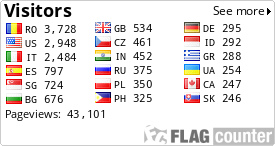## joi, 18 martie 2010

6 w / 0 l / 0 c -> +11.28 m.u. (inv.39 m.u. -> 28.92% ROI)

DNB stands, for those who doesn't know, Draw No Bet, meaning that if the match ended in a draw in regular time the bet on 1 or 2 is cancelled. It is the same thing as AH 0 or AH 0:0.

Some bookies do not offer this kind of bet explicitly so I will show you some maths which will help you to get DNB manually. These computational formula will also help you to see if the bookie displays a correct DNB odd in case it offers such kind of bet.

Let's suppose you want to bet 1 on a match between A and B team (soccer). Total stack involved let it be S. The odds for 1 - O1 and for x - O2. So you will split S in S1 and S2, S1 - stack to bet on 1 and S2 stack to bet on x.

Initial equations:

S1 + S2 = S
S1 * O1 - S2 = P (profit in case the match ends in 1)
S2 * O2 - S1 = S (return of initial stack in case the match ends in x)

Computations:

S1 = S - S2
S2 * O2 - (S - S2) * O1 = S ->
S2 * O2 - S * O1 + S2 * O1 = S ->
S2 * (O1 + O2) - S * O1 = S ->
S2 * (O1 + O2) = S + S * O1 ->
S2 * (O1 + O2) = S * (1 + O1) ->

S2 = S * (1 + O1) / (O1 + O2) (final formula)

S1 = S - S2 ->
S1 = S - S * (1 + O1) / (O1 + O2) ->

S1 = S * (1 - (1 + O1) / (O1 + O2)) (final formula)

Knowing both S1 and S2, you can determine the profit in case the match ends in 1:

S1 * O1 - S2 * O2 = P

More than that, knowing the profit, you can determine easily the odd for DNB O(dnb):

O(dnb) = (S + P) / S ->

O(dnb) = 1 + (P / S) (final formula depending on S and P)

In case you want to compute O(dnb) no matter S and P, the formula will be:

O(dnb) = 1 + ((S1 * O1 - S2) / S) ->
O(dnb) = 1 + (S * (1 - (1 + O1) / (O1 + O2)) - S * ((1 + O1) / (O1 + O2)))) / S) ->
O(dnb) = 1 + (1 - (1 + O1) / (O1 + O2)) - ((1 + O1) / (O1 + O2)) ->
O(dnb) = 2 - 2 * ((1 + O1) / (O1 + O2)) ->
O(dnb) = 2 * (1 - (1 + O1) / (O1 + O2)) ->
O(dnb) = 2 * ((O1 + O2 - 1 - O1) / (O1 + O2)) ->

O(dnb) = 2 * (O2 - 1) / (O1 + O2) (final formula NOT depending on S and P)

The last one is the most important final formula from all.

These formula can be of course applied if you want to bet on team B using this kind of bet (DNB). Just change the O1 accordingly with the odd for 2...

Online Users LCtest